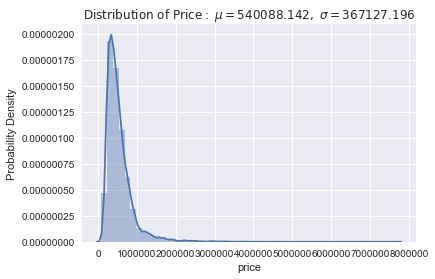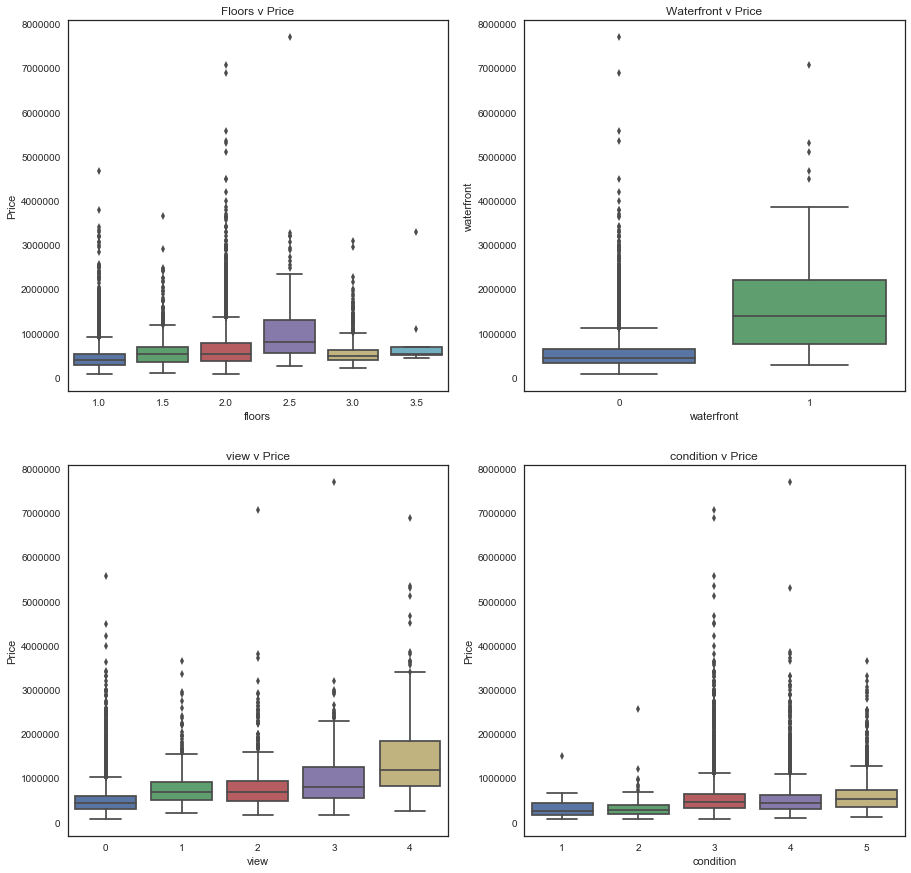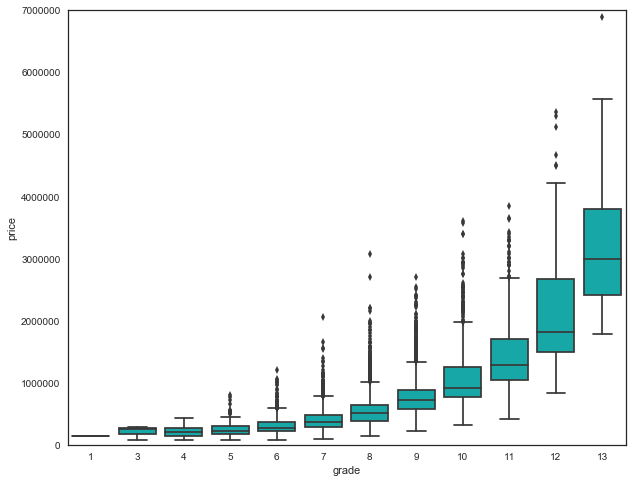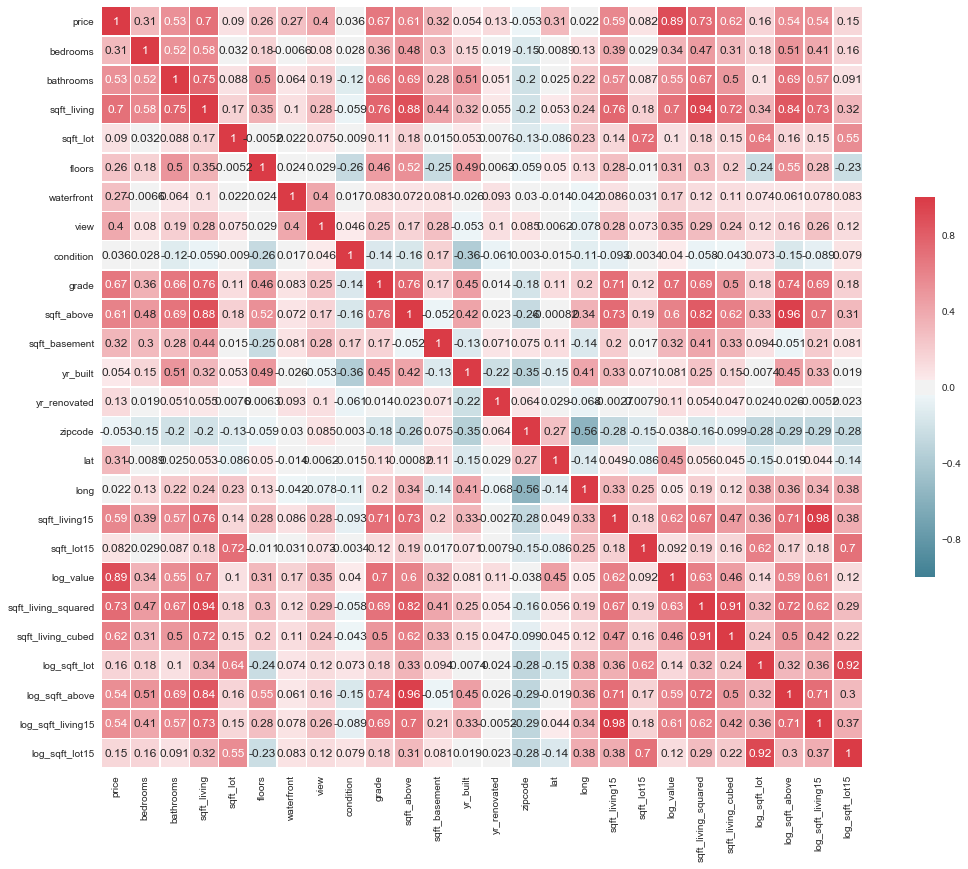Francisca Dias

## Introduction¶

For this analysis I will use the proportion of response variance accounted for as a criterion for evaluating regression performance in the King County housing study. This criterion is also known as the coefficient of determination or R-squared.

We compute its value in the test set by squaring the correlation of observed and predicted response values.

The dataset represents 21,613 King County Houses. We later split the data into 14,491 training observations and 7,122 test observations.

Relationships between pairs of variables in this problem are shown in the correlation heatmap, which orders explanatory variables (features) by their correlation with the response variable, the log Price, in US Dollars.

From the correlation heatmap we can see the importance of sqft_living and grade in explaining housing values.

When a response variable is positively skewed (as is the median value of homes), regressing the log of the response on the linear predictor often provides a better fitting model than regressing the original response on the linear predictor. That is the reason why our response variable, price, will be converted to log.

A more closer look at the correlation heatmap also reveals strong correlations among predictors.

Correlations among predictors is called multicollinearity and we should pay attention in regression contexts.

I used a model, called "my_model" where I included a polynomial regression. Therefore I can introduce the concept of multicollinearity by using the square and cube of a sqft_living.

This model, which will serve as a baseline for comparing other regression models in this problem, explains 52 percent of response variance in the training set and the same 52 percent in the test set. The response in this problem is the natural logarithm of home prices.

I will analyse various regression models.

I begin with tree-structured regression using the some of the features that I consider relevant. This model fits the training and test set well, explaining 55 percent of response variance in the training set and 53 percent on the test set.

If I include the set of variables from the original model plus a few log variables, to tree-structured regression, the model performs better, by explaining 57 percent of response variance in the training set, and 55 percent in the test set.

Finally I approach the Random Forest that is an ensemble learning method for classification and regression (our case). Fitting the data from the original model, it performs very well on the training set, explaining 91 percent of response variance, but explains only 50 percent on the test set. This big gap suggest over-fitting.

If I include the set of variables from the original model plus a few log variables into the Random Forest it makes a big difference on the test set, where now we get 93 percent on the training set and 59 percent on the test set.

This dataset can be found here.

## Libraries¶

In :
import warnings
warnings.filterwarnings("ignore")
from __future__ import division, print_function
import pandas as pd
from pandas.tools.plotting import scatter_matrix
import numpy as np  # arrays and math functions
from scipy.stats import uniform  # for training-and-test split
import statsmodels.api as sm  # statistical models (including regression)
import statsmodels.formula.api as smf  # R-like model specification
from sklearn.tree import DecisionTreeRegressor  # machine learning tree
from sklearn.ensemble import RandomForestRegressor # ensemble method
pd.set_option('display.float_format', lambda x: '%.3f' % x)


In :
houses_df['idx'] = range(len(houses_df))  # for use as index
houses = houses_df.set_index(['idx'])

In :
houses.columns

Out:
Index(['id', 'date', 'price', 'bedrooms', 'bathrooms', 'sqft_living',
'sqft_lot', 'floors', 'waterfront', 'view', 'condition', 'grade',
'sqft_above', 'sqft_basement', 'yr_built', 'yr_renovated', 'zipcode',
'lat', 'long', 'sqft_living15', 'sqft_lot15'],
dtype='object')
In :
del houses["id"]

In :
del houses["date"]

In :
houses.columns

Out:
Index(['price', 'bedrooms', 'bathrooms', 'sqft_living', 'sqft_lot', 'floors',
'sqft_basement', 'yr_built', 'yr_renovated', 'zipcode', 'lat', 'long',
'sqft_living15', 'sqft_lot15'],
dtype='object')

## Missing Data¶

In :
total = houses.isnull().sum().sort_values(ascending=False)
percent = (houses.isnull().sum()/houses.isnull().count()).sort_values(ascending=False)
missing_data = pd.concat([total, percent], axis=1, keys=['Total', 'Percent'])

Out:
Total Percent
sqft_lot15 0 0.000
condition 0 0.000
bedrooms 0 0.000
bathrooms 0 0.000
sqft_living 0 0.000
sqft_lot 0 0.000
floors 0 0.000
waterfront 0 0.000
view 0 0.000
sqft_living15 0 0.000
sqft_above 0 0.000
sqft_basement 0 0.000
yr_built 0 0.000
yr_renovated 0 0.000
zipcode 0 0.000
lat 0 0.000
long 0 0.000
price 0 0.000

There are no missing values in this dataset.

## Target Variable¶

Let´s have a first look of the main statistics of the variable we are trying to predict: house prices.

In :
houses['price'].describe()

Out:
count     21613.000
mean     540088.142
std      367127.196
min       75000.000
25%      321950.000
50%      450000.000
75%      645000.000
max     7700000.000
Name: price, dtype: float64

There is some informartion we can interpret here:

• The mean value of homes of 540,088 is greater than the median of 450,000. This is an indication that the response variable is positively skewed. When this happens, regressing the log of price usually provides a better fitting, compared to the original price;
• The maximum house price is listed as 7,700.000 and the minimum price is 75,000.
In :
import seaborn as sns
import matplotlib.pyplot as plt
%matplotlib inline

mu = houses['price'].mean()
sigma = houses['price'].std()
plt.xlabel('House Price')
plt.ylabel('Probability Density')

plt.title(r'$\mathrm{Distribution\ of\ Price:}\ \mu=%.3f,\ \sigma=%.3f$'%(mu,sigma))
sns.distplot(houses['price']);## Numerical Features¶

In :
houses.grade.value_counts()

Out:
7     8981
8     6068
9     2615
6     2038
10    1134
11     399
5      242
12      90
4       29
13      13
3        3
1        1
Name: grade, dtype: int64

Instead of describing all statistical attributes of features, it would be easier to plot them in boxplots. They will give us the median, lower and upper quartile and outliers for each values that these features take.

Let´s see the distributions between the following features: floors, waterfront, view, condition and grade.

In :
# Suplots of categorical features v price

sns.set_style('white')
f, axes = plt.subplots(2,2, figsize = (15,15))

# Plot [0,0]
sns.boxplot(data = houses, x = 'floors', y = 'price', ax = axes[0,0])
axes[0,0].set_xlabel('floors')
axes[0,0].set_ylabel('Price')
axes[0,0].set_title('Floors v Price')

# Plot [0,1]
sns.boxplot(x = 'waterfront', y = 'price', data = houses, ax = axes[0,1])
axes[0,1].set_xlabel('waterfront')
axes[0,1].set_ylabel('waterfront')
axes[0,1].set_title('Waterfront v Price')

# Plot [1,0]
sns.boxplot(x = 'view', y = 'price', data = houses, ax = axes[1,0])
axes[1,0].set_xlabel('view')
axes[1,0].set_ylabel('Price')
axes[1,0].set_title('view v Price')

# Plot [1,1]
sns.boxplot(x = 'condition', y = 'price', data = houses, ax = axes[1,1])
axes[1,1].set_xlabel('condition')
axes[1,1].set_ylabel('Price')
axes[1,1].set_title('condition v Price');#### Insights¶

• Median prices for houses tend to increase as the number of floors per house increases. We can also see outliers, specially in houses with two floors.
• Median prices of houses with a waterfront is higher than with no waterfront. A house with a waterfront appears to have larger variability in price.
• Median prices of houses tend to be the same whether there´s one, two or three views. Houses with 4 views have a greater median and also more variability in price.
• When it comes to condition, median tend to be the same across all 3, 4 and 5 values.

The feature Grade takes on more values, therefore I will plot this variable in a another graph.

In :
sns.set(style="white")
f, ax = plt.subplots(figsize=(10, 8))
fig = sns.boxplot(x='grade', y="price", data=houses, color="c")
fig.axis(ymin=0, ymax=7000000);• As we expected, as the house grade increases, its price also increases.
• This boxplot is useful to check the outliers in prices too. We said in the beginning that the maximum price lited was worth 7 Million, and we can see it on the last box, as an outlier.
• Also the variability in price increases as house grade increases.
In :
### floors, waterfront, view, condition, grade not log
### more correlated :


## Log of Features¶

In :
# computed variables for linear model used by my Model
houses['log_value'] = np.log(houses['price'])

houses['sqft_living_squared'] = np.power(houses['sqft_living'], 2)
houses['sqft_living_cubed'] = np.power(houses['sqft_living'], 3)

houses['log_sqft_lot'] = np.log(houses['sqft_lot'])
houses['log_sqft_above'] = np.log(houses['sqft_above'])
houses['log_sqft_living15'] = np.log(houses['sqft_living15'])
houses['log_sqft_lot15'] = np.log(houses['sqft_lot15'])


## Heatmap¶

In :
import seaborn as sns
import matplotlib.pyplot as plt
%matplotlib inline

sns.set(style="white")
# Compute the correlation matrix
corr = houses.corr()

f, ax = plt.subplots(figsize=(19, 14))
cmap = sns.diverging_palette(220, 10, as_cmap=True)

sns.heatmap(corr, annot=True, cmap=cmap, center=0,
square=True, linewidths=.5, cbar_kws={"shrink": .5});## Constructing three models¶

In :
# structure of my model for baseline for comparisons
my_model = 'log_value ~ sqft_living + sqft_living_squared + \
sqft_living_cubed + log_sqft_lot + log_sqft_above + log_sqft_living15 + \
log_sqft_lot15 '

In :
# for comparison lets look at a simple model with the original variables
simple_model = 'log_value ~ bedrooms + bathrooms + grade + sqft_above + \
sqft_living15 '

In :
# original variables plus variables that add value for trees
# that is... variables that are not simple monotonic transformations
# of the original explanatory variables
full_model = 'log_value ~ bedrooms + bathrooms + grade + sqft_above + sqft_living15 + \
log_sqft_lot + log_sqft_above + log_sqft_living15 + log_sqft_lot15'


I will split the data into training and test by creating a new column "runiform" that will take values, randomly, from 0 to 1. Then I am imposing a rule in which values above 0.33 wil belong to my train dataset, and values below will be to test set.

Each dataset will still have 21 features, but train set will gave 14491 observations, whereas test will have only 7122 observations.

## Split / Test the dataset¶

In :
# employ training-and-test regimen for model validation
np.random.seed(4444)
houses['runiform'] = uniform.rvs(loc = 0, scale = 1, size = len(houses))
houses_train = houses[houses['runiform'] >= 0.33]
houses_test = houses[houses['runiform'] < 0.33]
# check training data frame
print('\nhouses_selected_train data frame (rows, columns): ',houses_train.shape)
# check test data frame
print('\nhouses_selected_test data frame (rows, columns): ',houses_test.shape)

houses_selected_train data frame (rows, columns):  (14491, 27)
price  bedrooms  bathrooms  sqft_living  sqft_lot  floors  \
idx
0    221900.000         3      1.000         1180      5650   1.000
2    180000.000         2      1.000          770     10000   1.000
3    604000.000         4      3.000         1960      5000   1.000
4    510000.000         3      2.000         1680      8080   1.000
5   1225000.000         4      4.500         5420    101930   1.000

waterfront  view  condition  grade    ...     sqft_living15  sqft_lot15  \
idx                                        ...
0             0     0          3      7    ...              1340        5650
2             0     0          3      6    ...              2720        8062
3             0     0          5      7    ...              1360        5000
4             0     0          3      8    ...              1800        7503
5             0     0          3     11    ...              4760      101930

log_value  sqft_living_squared  sqft_living_cubed  log_sqft_lot  \
idx
0       12.310              1392400         1643032000         8.639
2       12.101               592900          456533000         9.210
3       13.311              3841600         7529536000         8.517
4       13.142              2822400         4741632000         8.997
5       14.018             29376400       159220088000        11.532

log_sqft_above  log_sqft_living15  log_sqft_lot15  runiform
idx
0             7.073              7.200           8.639     0.943
2             6.646              7.908           8.995     0.445
3             6.957              7.215           8.517     0.638
4             7.427              7.496           8.923     0.521
5             8.266              8.468          11.532     0.900

[5 rows x 27 columns]

houses_selected_test data frame (rows, columns):  (7122, 27)
price  bedrooms  bathrooms  sqft_living  sqft_lot  floors  \
idx
1   538000.000         3      2.250         2570      7242   2.000
6   257500.000         3      2.250         1715      6819   2.000
7   291850.000         3      1.500         1060      9711   1.000
8   229500.000         3      1.000         1780      7470   1.000
10  662500.000         3      2.500         3560      9796   1.000

waterfront  view  condition  grade    ...     sqft_living15  sqft_lot15  \
idx                                        ...
1             0     0          3      7    ...              1690        7639
6             0     0          3      7    ...              2238        6819
7             0     0          3      7    ...              1650        9711
8             0     0          3      7    ...              1780        8113
10            0     0          3      8    ...              2210        8925

log_value  sqft_living_squared  sqft_living_cubed  log_sqft_lot  \
idx
1       13.196              6604900        16974593000         8.888
6       12.459              2941225         5044200875         8.827
7       12.584              1123600         1191016000         9.181
8       12.344              3168400         5639752000         8.919
10      13.404             12673600        45118016000         9.190

log_sqft_above  log_sqft_living15  log_sqft_lot15  runiform
idx
1             7.682              7.432           8.941     0.123
6             7.447              7.713           8.827     0.257
7             6.966              7.409           9.181     0.192
8             6.957              7.484           9.001     0.182
10            7.528              7.701           9.097     0.272

[5 rows x 27 columns]

In :
# examine the correlations across the variables before we begin modeling
houses_train_df_vars = houses_train.loc[ : ,['log_value', 'bedrooms','condition', 'grade', 'log_sqft_lot', 'log_sqft_living15']]
print(houses_train_df_vars.corr())

                   log_value  bedrooms  condition  grade  log_sqft_lot  \
log_value              1.000     0.337      0.038  0.704         0.145
bedrooms               0.337     1.000      0.021  0.347         0.184
condition              0.038     0.021      1.000 -0.151         0.078
grade                  0.704     0.347     -0.151  1.000         0.188
log_sqft_lot           0.145     0.184      0.078  0.188         1.000
log_sqft_living15      0.607     0.400     -0.092  0.684         0.370

log_sqft_living15
log_value                      0.607
bedrooms                       0.400
condition                     -0.092
log_sqft_lot                   0.370
log_sqft_living15              1.000

In :
houses.columns

Out:
Index(['price', 'bedrooms', 'bathrooms', 'sqft_living', 'sqft_lot', 'floors',
'sqft_basement', 'yr_built', 'yr_renovated', 'zipcode', 'lat', 'long',
'sqft_living15', 'sqft_lot15', 'log_value', 'sqft_living_squared',
'sqft_living_cubed', 'log_sqft_lot', 'log_sqft_above',
'log_sqft_living15', 'log_sqft_lot15', 'runiform'],
dtype='object')

## Pairplot¶

In :
#scatterplot

sns.set()

sns.pairplot(houses_train_df_vars, size = 2.5)
plt.show();